# Stack Data Structure

Stack is one of the most powerful and most useful concept in programming. It is an ordered collection of items where items can be inserted and deleted from the same end. Only one end of the stack is accessible while the other is restricted.

The end of the stack where the insertion and deletion of items take place is called the Top of the stack. The last item to be added onto the stack is the first item to be deleted. So, a stack is also called a Last In First Out (LIFO) list.

For example, A stack of books. We add new books on top of old ones and the topmost book is removed first.Figure: A stack of books

A general example of a stack is as shown in the fig below.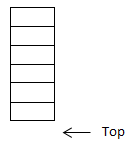Figure: An empty stackFigure: A full stack

## Operations in a Stack

### PUSH operation

When a new item is added on a stack, an item is pushed on the stack. The new item is added on the top of the stack.

Example: Push operation in a stack

• We have a stack S containing 4 elements as 1, 2, 3 and 4.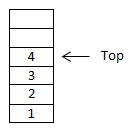• The operation push(s, x) pushes the item x at the top of the stack S. If we push item 5 on to the stack then the resulting stack contains 1, 2, 3, 4 and 5.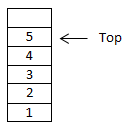• Again, if we push an item 6 onto the stack, the resulting stack contains 1, 2, 3, 4, 5 and 6.• Now, this stack is full. A push operation cannot be performed on a full stack as there is no vacant space to place the item on the stack.
• The result of attempting to push an item onto the top of a full stack is called Stack Overflow.

### POP operation

When an item is removed from the stack, the item is popped from the stack. The item to be popped is the item at the top of the stack.

The operation pop(s) removes the item at the top of stack and returns it as a functional value meaning that the operation

`i = pop (S)`

removes the item currently at the top of stack S and assigns the value that has just been popped to variable i.

Example: Pop operation in a stack

• We have a stack S with 2 elements 1 and 2.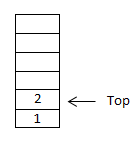• If we perform the pop operation, 2 is popped from the stack and the resulting stack contains only one element 1.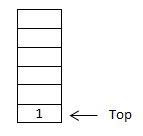• If we perform the pop operation again then the resulting stack contains no element.• Here, the resulting stack cannot be popped because the stack is empty meaning that there are no elements to pop.
• The result of attempting to pop an item from the top of an empty stack is called Stack Underflow.

### createEmptyStack operation

This operation is used to create an empty stack.

### isFull operation

This operation is used to determine whether the stack is full or not.

### isEmpty operation

This operation is used to determine whether the stack is empty or not.

### stackTop operation

Another operation that can be implemented on a stack is to determine what the top item on the stack is without removing it. This operation is used to determine the top item currently on the stack but does not remove it.

## Stack as an Abstract Data Type (ADT)

Since the following operations of stack can be implemented by using a data structure so stack is an Abstract Data Type (ADT):

Operations Description
CreateEmptyStack (S) This operation is used to create an empty stack S.
Push (S, x) This operation is used to add an item x on to the top of the stack S. This operation pushes the item if and only if the stack has an empty or vacant space.
Pop (S) This operation is used to remove the item currently at the top of stack. This item pops the item at the top of stack if and only if the stack is not empty.
stackTop (S) This operation returns the item currently at the top of the stack if and only if the stack is not empty.
isFull (S) This operation is used to determine whether the stack S is full or not. It returns true value (i.e. 1) if the stack is full otherwise it returns false.
isEmpty (S) This operation is used to determine whether the stack S is empty of not. It returns true value (i.e.0) if the stack is empty otherwise it returns false.

## Implementation of Stack

A stack can be implemented in two ways:

1. Static Implementation or Array implementation

2. Dynamic Implementation or Linked List implementation

### 1. Array implementation of Stack

In array implementation of stack,

• we use a one dimensional array which is large enough to store the data or items for maximum size of the stack.
• We also use an integer value top which is used to indicate the top of the stack.
• We declare a structure in which
• One member is an array of a type of data to be stored in stack named items.
• Another member is top which is an integer data type and is used to keep track of the current top item on the stack.
• The top is incremented and decremented as items are added and deleted respectively on the stack.

Creating an Empty Stack – createEmptyStack Function

To create an empty stack,

• We initialize top as a unique value to indicate the empty stack.
• Commonly, for better understanding, we initialize top as 0 or -1.
• Here, we initialize top as -1.
• The data will be stored from array index location 0 into the array items.
```being procedure createEmptyStack
top ← 1```

isEmpty Function

When the stack is empty,

• The value of top is -1.
• This function returns true value (i.e. 1) if the stack is empty, otherwise it returns false (i.e. 0).
```begin procedure isEmpty
if top equals to -1
return 1
else
return 0```

isFull Function

When the stack is full,

• the value of top is MAX-1 as the index of array starts from 0 and goes to maximum number of items that the stack can store i.e. MAX-1.
• This function returns true i.e. 1 if the stack is full, otherwise it returns false (i.e. 0).
```begin procedure isFull
if top equals to MAX -1
return 1
else
return 0```

PUSH Function

To push an item,

• Before adding the item to the top of the stack, we check whether the stack is full or not by seeing the result of the isFull function defined above.
• If the stack is full, the isFull function returns true value and no item can be added.
• If the stack is not full, the isFull function returns false and then we increment the top by 1 and add the item on to the top of stack.
```begin procedure push: stack, newItem
if top equals to MAX -1
print "Stack is Full / Stack Overflow" and exit.
return 0
else
top ← top + 1
stack[top] ← newitem```

POP Function

To pop an item,

• Before removing an item from the top of the stack, we check whether the stack is empty or not by seeing the result of the isEmpty function defined above.
• If the stack is empty then the isEmpty function returns true value and no item can be removed from the stack.
• If the stack is not empty then the isEmpty function returns false value and we remove the top item from the stack by decrementing top by 1.
```begin procedure pop: stack
if top less than 0
print "Stack is Full / Stack Overflow" and exit.
return 0
else
poppedItem ← stack[top]
top ← top - 1
return poppedItem
```

### Example: C Program to implement stack data structure using Array.

```#include<stdio.h>
#include<conio.h>
#include<stdlib.h>

#define MAX 10

struct stack
{
int items[MAX];
int top;
};
typedef struct stack st;

void createemptystack(st *s)
{
s->top=-1;
}

int isfull(st *s)
{
if (s->top==MAX-1)
return 1;
else
return 0;
}

int isempty(st *s)
{
if (s->top==-1)
return 1;
else
return 0;
}

void push(st *s)
{
int newitem;
printf("Enter item to be inserted: ");
scanf("%d",&newitem);
if (isfull(s))
{
printf("STACK FULL");
}
else
{
s->top++;
s->items[s->top]=newitem;
}
}

void display(st *s)
{
int i;
printf("n The items are: n");
for (i=s->top;i>=0;i--)
{
printf("n %d",s->items[i]);
}
}

void pop (st *s)
{
if (isempty(s))
{
printf("n STACK EMPTY n");
}
else
{
printf("Item popped= %d",s->items[s->top]);
s->top--;
}
}

void main()
{
int ch;
int loop;
loop=1;
st *s;

createemptystack(s);

do
{
printf("n ***STACK OPERATIONS");
printf("n 1. PUSH");
printf("n 2. DISPLAY");
printf("n 3. POP");
printf("n 4. EXIT");
printf("n ***************");
scanf("%d", &ch);

switch (ch)
{
case 1:
push(s);
break;
case 2:
display(s);
break;
case 3:
pop(s);
break;
case 4:
printf("THANK YOU");
loop=0;
exit(0);
default:
printf("Invalid choice");
}
} while(loop);

getch();
}```

## Application of Stack

A stack is used to:

• Convert decimal number into binary number

• Print characters or strings in reverse order

• Evaluate prefix and postfix expressions

• Check balance of parenthesis in expressions

• Retain the page-visited history in a web browser

• Store the sequence of undo operations in text editor

• Create auxiliary data structure for algorithms

• Create component of other data structure